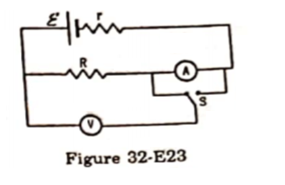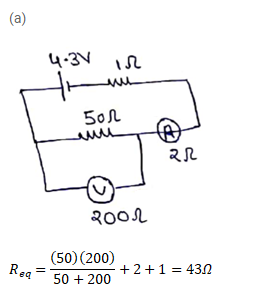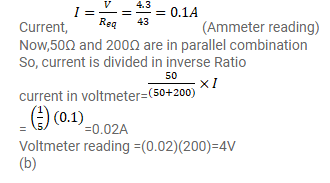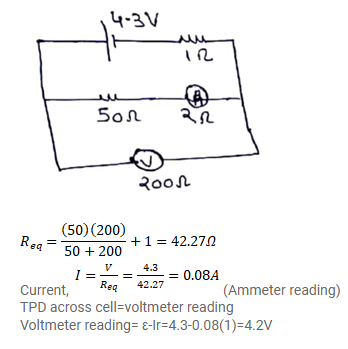# The emf ε and the internal resistance r of

Question:

The emf $\varepsilon$ and the internal resistance r of the battery shown in figure (32-E23) are $4.3 \mathrm{~V}$ and $1.0 \Omega$ respectively. The external resistance $R$ is $50 \Omega$. The resistances of the ammeter and voltmeter are $2.0 \Omega$ and $200 \Omega$ respectively.

(a) Find the readings of the two meters.

(b) The switch is thrown to the other side. What will be the readings of the two meters now?Solution: